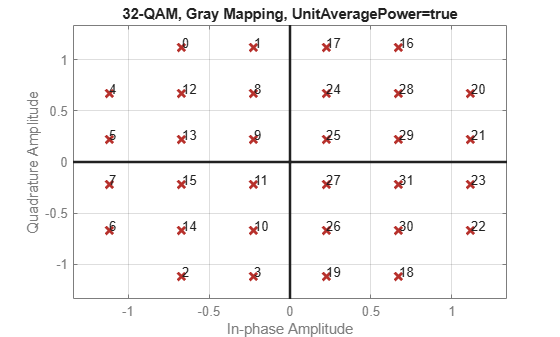Plot Signal Constellations

Create 16-PSK Constellation Diagram

This example shows how to plot a PSK constellation having 16 points.

Set the parameters for 16-PSK modulation with no phase offset and binary symbol mapping.

M = 16;             % Modulation alphabet size
phOffset = 0;       % Phase offset
symMap = 'binary';  % Symbol mapping (either 'binary' or 'gray')

Construct the modulator object.

pskModulator = comm.PSKModulator(M,phOffset,'SymbolMapping',symMap);

Plot the constellation.

constellation(pskModulator)Create 32-QAM Constellation Diagram

This example shows how to plot a QAM constellation having 32 points.

Use the qammod function to generate the 32-QAM symbols with binary symbol ordering.

M = 32;
data = 0:M-1;
sym = qammod(data,M,'bin');

Plot the constellation. Label the order of the constellation symbols.

scatterplot(sym,1,0,'b*');
for k = 1:M
text(real(sym(k))-0.4,imag(sym(k))+0.4,num2str(data(k)));
end
axis([-6 6 -6 6])Create 8-QAM Gray Coded Constellation Diagram

Use the qammod function to generate the 8-QAM symbols with Gray symbol ordering. Note that Gray coding is the default symbol mapping for the qammod function.

M = 8;
data = 0:M-1;
sym = qammod(data,M);

Plot the constellation. Label the order of the constellation symbols.

scatterplot(sym,1,0,'r*');
grid on
for k = 1:M
text(real(sym(k))-0.4,imag(sym(k))+0.4,num2str(data(k)));
end
axis([-4 4 -2 2])Plot a Triangular Constellation for QAM

This example shows how to plot a customized QAM reference constellation.

Describe the constellation.

inphase = [1/2 -1/2 1 0 3/2 -3/2 1 -1];
quadr = [1 1 0 2 1 1 2 2];
inphase = [inphase; -inphase];
inphase = inphase(:);

Construct a constellation diagram System object using name-value pairs to specify the title, the axes limits, the reference marker type, and the reference marker color.

constDiagram = comm.ConstellationDiagram('Title','Customized Constellation for QAM', ...
'XLimits',[-3 3],'YLimits',[-3 3], ...
'ReferenceConstellation',refConst, ...
'ReferenceMarker','*','ReferenceColor',[0 1 0]);

Plot the customized constellation.

constDiagram(refConst)